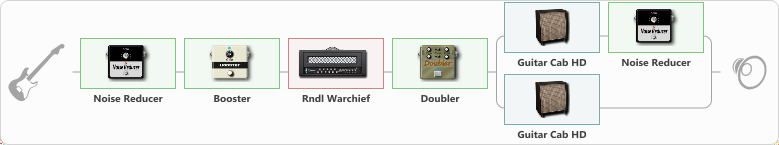# Mount for war Metallic 80s sound

Discussion in 'ToneLib-GFX presets' started by metal of puppets, Mar 2, 2022.

1. Mount for war Metallic 80s sound

Preset name: Mouth for war

Mount for war Metallic 80s sound

Effects chain:Effect: "Noise Reducer" (Dynamics / Filter), active - "yes"
{
"Sens" = 50
"Mode" = Soft
}

Effect: "Booster" (Dynamics / Filter), active - "yes"
{
"Gain" = 100
}

Effect: "Rndl Warchief" (Amp simulators), active - "yes"
{
"Gain" = 90
"Bass" = 80
"Middle" = 80
"Treble" = 100
"Presence" = 100
"Master" = 50
"Level (dB)" = 1
}

Effect: "Doubler" (Dynamics / Filter), active - "yes"
{
"A-Dly" = 21
"A-Inv" = On
"A-Level" = 80
"B-Dly" = 100
"B-Inv" = Off
"B-Level" = 80
}

Effect: "Splitter" (Dynamics / Filter)
{
"A-Bypass" = Off
"A-Pan" = -25
"A-Level" = 55
"B-Bypass" = Off
"B-Pan" = 25
"B-Level" = 55
"Width" = 30

'A' branch:
{

Effect: "Guitar Cab HD" (Cabinets), active - "yes"
{
"Model" = Peavey 5150 (4x12")
"Mic Model" = Ribbon M160
"Mic Position" = Center
"Mic Distance" = Middle
"Low Cut (Hz)" = 80
"Hi Cut (kHz)" = 20.0
"Mix" = 100
"Level (dB)" = 0
}

Effect: "Noise Reducer" (Dynamics / Filter), active - "yes"
{
"Sens" = 100
"Mode" = Hard
}
}
'B' branch:
{

Effect: "Guitar Cab HD" (Cabinets), active - "yes"
{
"Model" = Orange PPC (4x12")
"Mic Model" = Dynamic MD421
"Mic Position" = Middle
"Mic Distance" = Far
"Low Cut (Hz)" = 80
"Hi Cut (kHz)" = 20.0
"Mix" = 100
"Level (dB)" = 0
}
}
}

Note: You will need to download and install the ToneLib-GFX software to use the preset.

File size:
800 bytes
Views:
1,905